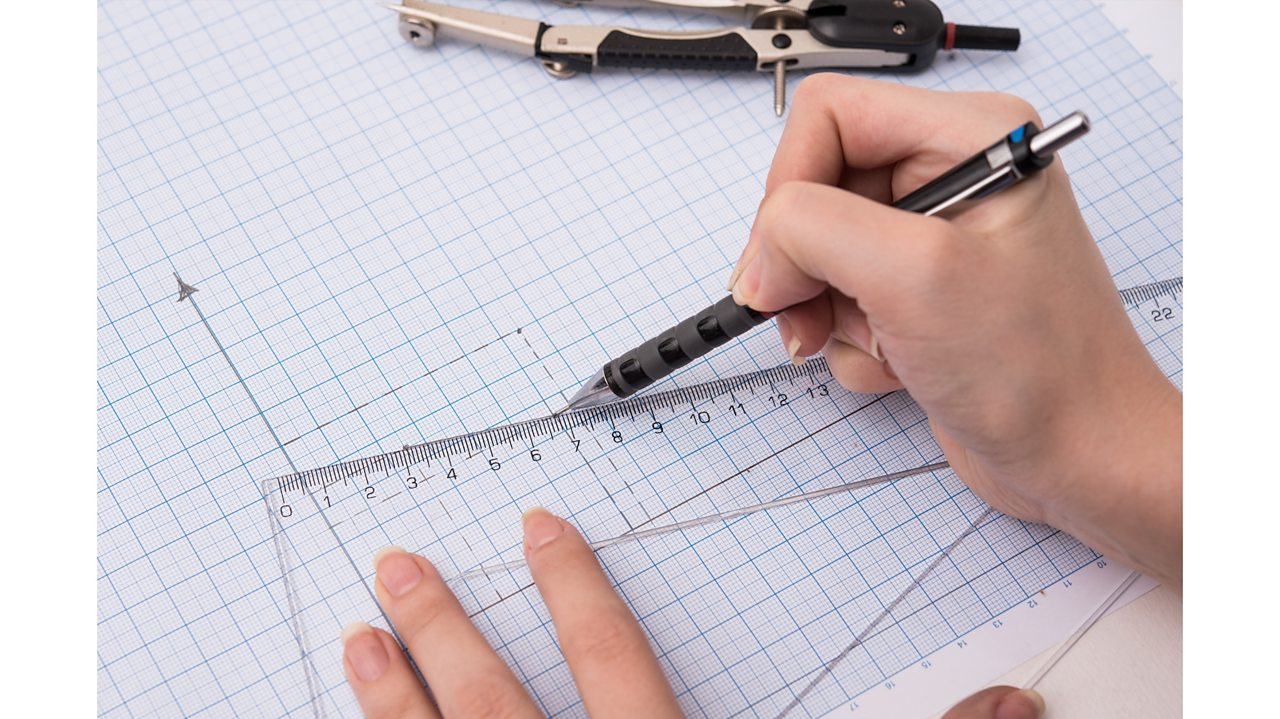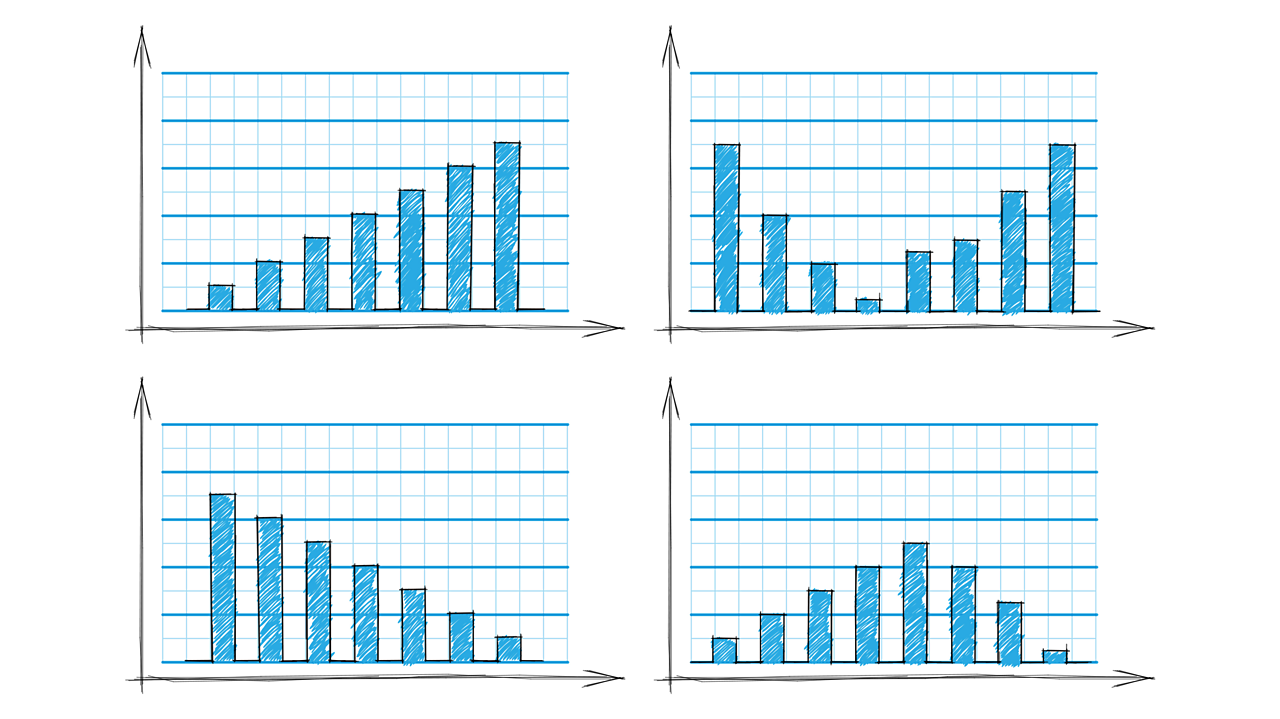# Graphs and charts

## Key points

• Different types of graphs and charts are needed to present results from experiments.
• Graphs and charts need to be drawn and labelled correctly.
• Use patterns in the data to reach a conclusion in an experiment.

True or false?

It is easier to see patterns in data from a graph than a table.

True.

Many people find graphs or charts much easier to understand. They can often see patterns or trends that are much harder to spot in tables of data.

## How to pick the correct chart

Watch this video on how to choose a graph or chart and how to draw them.

## Presenting data

After collecting and recording , scientists often put their data into a graph or a chart. This shows the data in a useful way and helps them to reach conclusions.

The most common ways of presenting data in science are and .

A line graph should be used when the independent and dependent variables are .

A bar chart should be used if the independent variable is .

or categoric data can also be shown on a pie chart. Pie charts are often used when using percentages of data to draw a graph.

If both the independent and dependent variables are continuous, how should data be presented?

With a line graph.

## Drawing graphs and charts

When drawing a chart or a graph, the independent variable goes on the horizontal (x) axis and the dependent variable goes on the vertical (y) axis.

Once this has been identified, follow these steps:

1. Choose scales for the axes so that the graph is as big as possible on the page.
2. Use a pencil and a ruler to draw axes, bars, or to .
3. Label the axes with the quantity and the unit, such as ‘Temperature, °C’.
4. Write a title for the graph.

True or false?

Units are not needed when drawing a graph.

False.

Units are important, so always include them because they give us the scale. Imagine if the race you wanted to run was in miles not metres. Or the time of a car journey was in days not hours.

## How to draw graphs and charts

Presenting data in a graph or chart needs to be done carefully. As well as the four steps described above, there are other things to think about.When drawing a line graph, it’s important to look at the range for the data before drawing a simple scale. When plotting the ‘Xs’ on the graph, the data must be presented accurately.1 of 3When drawing a bar chart, it’s best to see how many bars need to be drawn first. Look to see how big the tallest bar will be to help with the scale. If the independent variable is not continuous, leave a gap between each bar.2 of 3When drawing a pie chart, make sure the correct equipment is used. Use a pair of compasses and a protractor.3 of 3

True or false?

A gap should be left between bars on a bar chart if the data is not continuous.

True.

If the independent variable is not continuous, leave a gap between each bar.

## Analysing data

Finding patterns in data on a graph or chart is known as ‘interpreting relationships’. Plotting a chart or graph helps to show a pattern in the data - how the dependent variable depends on the independent variable.

Once points have been plotted for a line graph, draw a . The line should be drawn through as many points as possible, with equal numbers of points above and below the line. If there are any then these should be ignored when drawing the line of best fit.

Once the graph has been analysed, write a conclusion. Support a conclusion by saying what the graph shows, such as if there is a relationship between the two variables. A good way of describing the relationship is to say what happens to one variable as the other one increases or decreases. For example, the longer a kettle is left to boil, the hotter the water gets until it reaches 100°C.

### A step-by-step guide on drawing a line of best fit

What is the name of a result that should be ignored when drawing the line of best fit?

An outlier should be ignored when drawing a line of best fit.

## Analysing trends in graphs

When data is analysed and a relationship between two variables is described, it's important to say how changing one variable affects the other. These line graphs show some common shapes that arise when the independent variable (A) affects the dependent variable (B).

As A increases, B increases.

The yellow line is straight because each increase in A gives the same increase in B. This is also true in the straight parts of blue and red lines. When the blue line curves horizontally, the same increase in A gives a smaller increase in B. When the red line curves upwards, the same increase in A gives a greater increase in B.

As A increases, B decreases.

The yellow line is straight because each increase in A gives the same decrease in B. This is also true in the straight parts of blue and red lines. When the blue line curves downwards, the same increase in A gives a greater decrease in B. When the red line curves horizontally, the same increase in A gives a smaller decrease in B.

As A increases, B does not change.

The yellow line is straight because as A increases, there is no change in B.

On these graphs, which variable goes on the horizontal (x) axis?

The independent variable. The dependent variable goes on the vertical (y) axis.